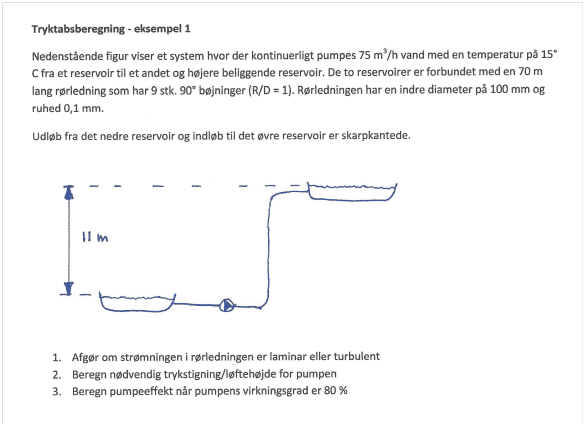# Pumping effect in a pipe system

• yellowbird321

## Homework Statement

Explain with basis in the figure how the necessary pumping effect is determined for a pump in a pipe system.We are given the following data:

T = 15 C

75 m3/h vand

H = 11 m

L = 70 m rørledning

D = 100 mm

R = έ = 0,1 mm

ή = 80 %

9 of 90 % standard elbows R/D = 1

P(15 C) = 9,94 Kj/m3

ή = (15 C) = 1,002 x 10-3 Pa s.

## Homework Equations

Calculation of incompressible fluids can be done by:

Δp12 = p2 – p1 = ρ(c22 – c12) / 2 + ρg(z2 – z1) + ρwdiss12

or

(u22 – u12) / 2 + g(z2 – z1) + P2 – P2 / ρ + W + F = 0.

## The Attempt at a Solution

[/B]
The first thing to do is to eliminate the kinetic energy. We have no kinetic energy because the diameter of the water tanks is so much larger than the water pipes. So we have:

g(z2 – z1) + P2 – P2 / ρ + W + F = 0

We still have potential energy. We do not have flow work, because each of the tanks is open to the atmosphere, så p – p is 0. So we have:

g(z2 – z1) + W + F = 0

We must calculate the work, og we have frictional effects.

With the given data, we can begin calculating pumping power.

The first we do is to determine the fluid velocity inside the pipe diameter.

Q = u Ac

u = 4Q / PI p2

= 4 x 75 m3/h / PI x (0,01 m)2

= 0,0095 m/s. Average velocity

We use the velocity in calculating Reynolds number

Rc = PuD / ή

= 9,94 Kj/m3 x 0,0095 m/s x 0,01 m / 1,002 x 10-3 Pa

= 0,942 < 4000.

The next step will be to calculate what the fanning friction factor is. Here a moody table ore fanning table, or the following can be used:

ζp = έ / D

= 0,001 m / 0,01 m

= 0,1 m .

When we find this factor, the next step will be to calculate what the frictional dissipation effects are.

F = 2f Fu2 (L / D)έ

First we calculate L / D. If we watch the figure, we see that there is a sudden compression, from the tanks large volume to the pipes smaller volume. We have 9 standard elbows, and then a sudden expansion. We have in other words a sudden compression, then a sudden expansion through the 9 standard 90’ere.

Lt = (70 m + 11 m) + 0,01 m (9(1))

= 81 m + 0,01 m (9(1))

(or alternative: 9 x 30, found in the table for standard 90 elbow, but the exercise says 1).

= 81,1 m. Total effektive lenght.

So solving for frictional dissipation

F = 2(ζ) (0,0095 m/s)2 (Lt/ D )

= 2(0,1) (0,0095 m/s)2 (81,1/ 0,01 )

= 2,23 x 10-9 m2 / s2.

We can proceed to solve for work now. We obtain the following:

-W = gΔz + F

= 9,82 m/s2 x (11 m) + 2,23 x 10-9

= 108 J/Kg.

So the negative work is the work put into the system, th positive work is that taken out of system. It is ideal work that has been calculated.

We was given the pump efficiently. So if we want to know the actual work, we do the following:

Wactual = WIdeal / ήpump

= -W / ήpump

= -108 / 80

= -2,25 J/Kg.

We are not done yet however. What we are interested in is power. The electrical power the pump requires to do the work

P = W p Q (power is always altid positive)

= 2,25 (9,98 Kg/m3) (75 m3/h)

= 1684 kW.

This is the electrical power needed to pump water from the left tank to the right tank.

Is this correct.

#### Attachments

You calculated the velocity incorrectly. Check the units.

You calculated the velocity incorrectly. Check the units.

Hi!

Thanks for the input. You mean, instead of using

Q = u Ac

u = 4Q / PI p2

= 4 x 75 m3/h / PI x (0,01 m)2

= 0,0095 m/s,

A = PI D2 / 4 = PI x (0,01)2 / 4 = 7,85 x 10-2 m2

u = (75 / 3600) x 1 / (7,85 x 10-2) = 2,65 x 10-4 m/s.

Estimate your answers when using a calculator. How can a 100 mm pipe have an area of 9 dm2 ?
75000 dm3/h is approximately 2 dm3/s so your answer should be of the order of magnitude of 2 m/s !

[edit: oops] 75000/3600 ##\approx## 20 dm3/s (not 2).
Divide by ##\approx## 1 dm2 to get ##\approx## 20 dm/s or 2 m/s -- at least the order of magnitude I mentioned was right.

##v## is 2.65 m/s

Last edited:
Hi!

Thanks for the reply. However, if Q is 75 m3/hr and the flow is pumped through a 0,01 m diameter pipe then:

A = PI D2 / 4

= PI x (0,01)2 / 4

= 7,85 x 10-5 m2

and

u = (750 / 3600) x 1 / (7,85 x 10-5)

= 2,65 x 10-8 m/s.

With the numbers given in the exersice, how can I get 2 m/s.

You got to do the arithmetic correctly. That 750 should be 75, and you're dividing by the area. I get 265 m/s, which is very high.

The pipe is 100 mm. That spells 0.1 m, not 0.01

u = (750 / 3600) x 1 / (7,85 x 10-5)

= 2,65 x 10-8 m/s.
Again, check what you do. Dividing by 10-5 can never get you something like 10-8